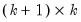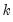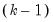testexog
Perform exogeneity (Granger causality) tests on a VAR.
Syntax
var_name.testexog(options)
Options

 name=arg Save the Wald test statistics in named matrix object. See below for a description of the statistics stored in the matrix. p Print output from the test.
The name= option stores the results in amatrix, whereis the number of endogenous variables in the VAR. In the firstrows, the i-th row, j-th column contains the Wald statistic for the joint significance of lags of the i-th endogenous variable in the j-th equation (note that the entries in the main diagonal are not reported in the table view). The degrees of freedom of the Wald statistics is the number of lags included in the VAR.
In the last row, the j-th column contains the Wald statistic for the joint significance of all lagged endogenous variables (excluding lags of the dependent variable) in the j-th equation. The degrees of freedom of the Wald statistics in the last row istimes the number of lags included in the VAR.
Examples
var var1.ls 1 6 lgdp lm1 lcpi
freeze(tab1) var1.testexog(name=exog)
The first line declares and estimates a VAR. The second line stores the exclusion test results in a named table TAB1, and stores the Wald statistics in a matrix named EXOG.
Cross-references
See “Diagnostic Views” for a discussion of other VAR diagnostics.